# 運動學¶

## 加速¶

Klipper總使用常加速度策略——列印頭的速度總是梯度變化到新的速度，而非使用速度突變的方式。Klipper著眼於列印件和列印頭之間的速度變化。離開擠出機的耗材十分脆弱，突然的移動速度和/或擠出流量突變可能會導致造成列印質量或床黏著能力的下降。甚至在無擠出時，如果列印頭和列印件頂端在同一水平面時，噴嘴的速度突變有可能對剛擠出的耗材進行剮蹭。限制列印頭相對於列印件的速度，可以減少剮蹭列印件的風險。

Klipper實現恒加速度控制，關鍵的方程如下：

``````velocity(time) = start_velocity + accel*time
``````

## 梯形發生器¶

Klipper 使用傳統的"梯形發生器"來產生每個動作的運動--每個動作都有一個起始速度，先恒定的加速度加速到一個巡航速度，再以恒定的速度巡航，最後用恒定的加速度減速到終點速度。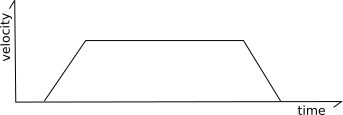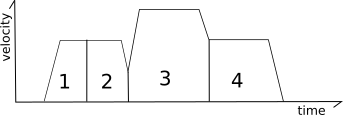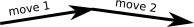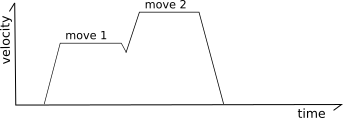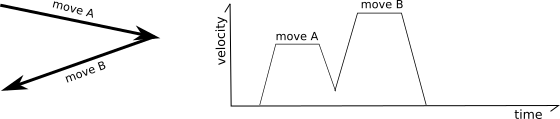``````end_velocity^2 = start_velocity^2 + 2*accel*move_distance
``````

### 預計算結果平滑¶

Klipper 實現了一種用於平滑短距離之字形移動的機制。參考以下移動：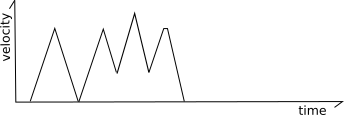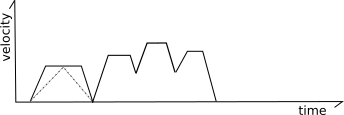## 生成步驟¶

Klipper 使用 iterative solver 為每個步進器生成步進時間。該代碼包含計算每個時刻頭部理想XYZ坐標的公式，並且它具有根據這些XYZ坐標計算理想步進器位置的動作公式。通過這些公式，Klipper 可以確定步進器應該在每個步進位置的理想時間。然後在這些計算的時間安排給定的步驟。

``````move_distance = (start_velocity + .5 * accel * move_time) * move_time
``````

``````move_distance = cruise_velocity * move_time
``````

``````cartesian_x_position = start_x + move_distance * total_x_movement / total_movement
cartesian_y_position = start_y + move_distance * total_y_movement / total_movement
cartesian_z_position = start_z + move_distance * total_z_movement / total_movement
``````

### XYZ 機型¶

``````stepper_x_position = cartesian_x_position
stepper_y_position = cartesian_y_position
stepper_z_position = cartesian_z_position
``````

### CoreXY 機型¶

``````stepper_a_position = cartesian_x_position + cartesian_y_position
stepper_b_position = cartesian_x_position - cartesian_y_position
stepper_z_position = cartesian_z_position
``````

### 三角洲機型¶

``````stepper_position = (sqrt(arm_length^2

- (cartesian_x_position - tower_x_position)^2
- (cartesian_y_position - tower_y_position)^2)
+ cartesian_z_position)
``````

### 擠出機動作¶

Klipper 在其自己的動作類別中實現了擠出機動作。由於每個打印頭移動的時間和速度對於每次移動都是完全已知的，因此可以獨立於打印頭移動的步進時間計算來計算擠出機的步進時間。

``````stepper_position = requested_e_position
``````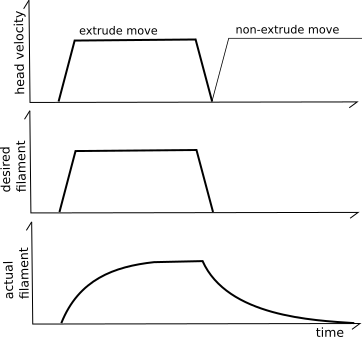"pressure advance"系統試圖通過使用不同型號的擠出機來解決這個問題。與其天真地相信每 mm^3 的長絲送入擠出機都會導致該量的 mm^3 立即離開擠出機，而是使用基於壓力的模型。當長絲被推入擠出機時壓力增加（如[胡克定律]（https://en.wikipedia.org/wiki/Hooke%27s_law）），擠出所需的壓力由通過噴嘴孔的流速決定（如 Poiseuille 定律）。關鍵思想是燈絲、壓力和流量之間的關係可以使用線性係數進行建模：

``````pa_position = nominal_position + pressure_advance_coefficient * nominal_velocity
``````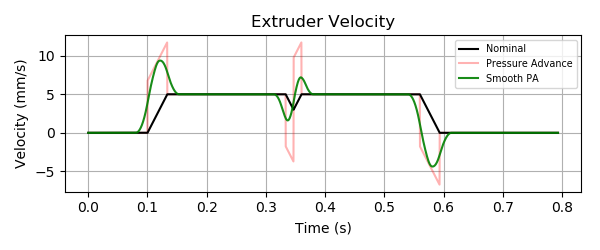“平滑”是使用擠出機位置在一小段時間內的加權平均值來實現的（由 `pressure_advance_smooth_time` 配置參數指定）。這種平均可以跨越多個 g 代碼移動。請注意擠出機電機將如何在第一次擠出移動的標稱開始之前開始移動，並將在最後一次擠出移動的標稱結束後繼續移動。

``````smooth_pa_position(t) =